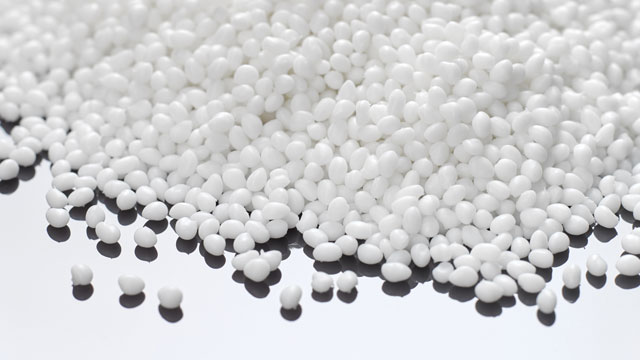Application note: Calculating mean particle size using sedimentation rate# Calculating mean particle size using sedimentation rateParticle size is a key parameter when producing particulate materials owing to its dominant effect on various utilisations of specific powders. Thus, particle size measurement is of high interest in many fields, such as cosmetic, chemistry, pharma, or food.

The MultiScan MS 20 from DataPhysics Instruments is a measuring device for automatic optical stability and aging analysis of liquid dispersions as well as the comprehensive characterisation of time- and temperature-dependent destabilisation mechanisms. It provides an easy and fast way to measure mean particle size in native dispersions. The mean particle size evaluation of two commercial polystyrene particles using an MS 20 device will be presented in this application note.

## Method and Technique

According to Stokes’ law, it is possible to calculate the mean particle diameter d from the sedimentation rate v :In which Δρ is the density difference between particle and liquid; g is Earth's gravitational acceleration of approximately 9.81 m/s2 and η is the viscosity of the liquid.

The MultiScan MS 20 from DataPhysics Instruments is a compact and versatile measuring device for stability analyses, since even the slightest changes in dispersions can be detected and evaluated. Thus, the MS 20 enables a fast and objective analysis of the sedimentation process, using DataPhysics’ so called migration front method. Additionally, for evaluating mean particle size, the MSC software integrated into the device is using the Richardson-Zaki equation  to correct the sedimentation rate due to volume fraction (concentration).

Therefore, MS 20 is an ideal device for evaluation of mean particle size.

Fig. 2: DataPhysics Instruments stability analysis system MultiScan MS 20 with six independent Scan Towers

## Experiment

Two commercial polystyrene particles (PS1 and PS2) with different particle sizes were used in this note (Table 1). The particle diameters of PS1 and PS2 particles given on the packages are around 20 µm and 40 µm, respectively. The solvent of the PS-suspensions is water, its density and viscosity are 0.998 g/cm3 and 1.002 mPas at room temperature.

20 ml of each polystyrene-suspension was poured in a transparent glass vial and measured at T = 25 °C every 1 min for 1 h. The measured zone is between 0 mm (bottom of the glass) and 57 mm (fill level of the vial).

Sample Particle diameter Particle density Particle volume concentration
PS1 ≅ 20 μm 1.06 g/cm3 5%
PS2 ≅ 40 μm 1.06 g/cm3 3%

Table 1: The properties of PS solutions

## Results

Figure 3 plots transmission and backscattering intensities against the position within the two polystyrene suspensions. The colour-coding of the curves indicates the time at which they were recorded, from red (first measurement, t = 0 s) to purple (last measurement, t = 1 h). Every curve represents an individual measurement.

The transmission and backscattering diagrams in Figure 3 show clearly time-dependent as well as position-dependent changes of the signal, indicating a typical sedimentation process. One can see that the changes in transmission and backscattering of PS1 are much slower than those of PS2, owing to the smaller particle size of PS1 particles.

With the respective function in the MSC software, the change of the migration front can be analysed utilising transmission and backscattering signals, resulting in an average sedimentation rate of 0.6262 mm/min for the PS1 suspension (Figure 4 left). According to the above theory, the mean particle diameter of PS1 can now be obtained by simply clicking on ‘calculate’ within the software (Figure 4 right). Note that the trigger line (intersection line) in the migration front method for measuring the sedimentation rate is usually set at the median of transmission or backscattering values (Figure 3, purple dotted line), in this case at Tr = 50% for PS1, to ensure the accuracy of the calculation for the mean particle diameter.

Accordingly, sample PS2 was analysed, leading to the sedimentation rate and mean particle size as displayed in Table 2. The particle diameters of PS1 and PS2 are 19.88 µm and 39.54 µm, respectively. Notably, the calculated particle diameters of the two samples are almost the same as the values given by the producer (Table 1), which confirms that is it possible to calculate mean particle diameter utilising the sedimentation rate.

Fig. 3: Intensity vs. position diagrams of transmission (top) and backscattering (bottom)

Sample Transmission sedimentation rate Transmission mean particle diameter Backscattering sedimentation rate Backscattering mean particle diameter
PS1 0.6262 mm/min 19.88 μm 0.6294 mm/min 19.93 μm
PS2 2.725 mm/min 39.54 μm 2.777 mm/min 39.91 μm

Table 2: Sedimentation rate and mean particle diameter evaluated based on transmission and backscattering signal

Furthermore, the mean particle diameters of PS1 and PS2 were calculated using backscattering signals by setting the trigger line at the median value of around 15 %. As shown in Table 2, the mean particle diameters calculated this way are around 20 µm (PS1) and 40 µm (PS2), which are similar to the results obtained using the transmission signals earlier. It can be concluded that the changes in both transmission and backscattering signals are able to be used for evaluating mean particle size utilising the sedimentation rate. Evidently, the above results underline the excellent applicability of MultiScan MS 20 not only to analyse stability issues, but also to calculate mean particle size with high validity.

Fig. 4: Evaluation of sedimentation rate and mean particle size for PS1

## Summary

Using the MultiScan MS 20 stability analysis system and its corresponding MSC software, an easy and fast way to evaluate mean particle size could be demonstrated. It has been proven that MS 20 can provide a valid measurement of mean particle sizes using the sedimentation rate, which is helpful in a wide range of research and industry fields, such as food, chemistry and pharma.